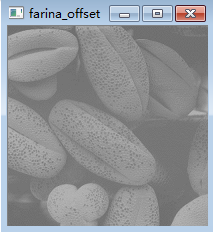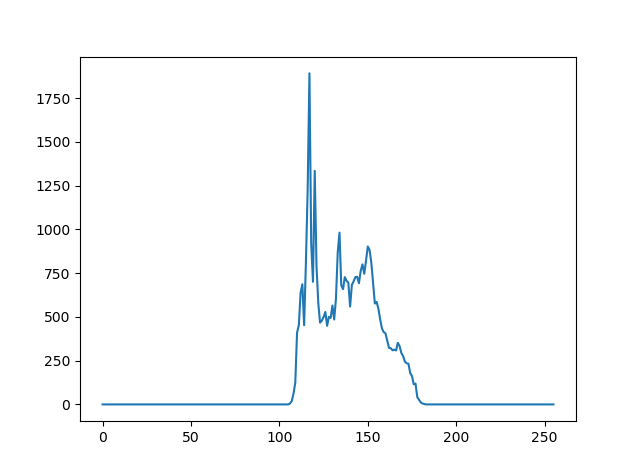# 图像增强之对比度拉伸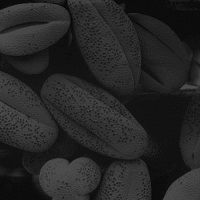import cv2
import matplotlib.pyplot as plt

farina = cv2.imread("farina.png", 0)

hist_full = cv2.calcHist([farina], , None, , [0, 256])

plt.plot(hist_full)
plt.show()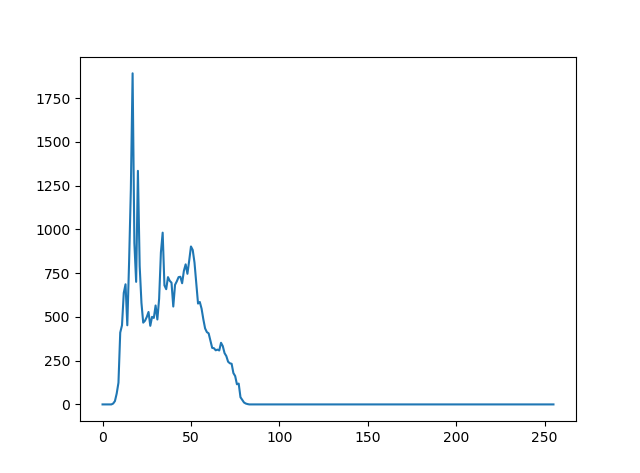$I\left(x,y）=\frac{I\left(x,y\right)-Imin}{Imax-Imin}\left(MAX-MIN\right)+MIN$

Imax = np.max(farina)
Imin = np.min(farina)
MAX = 255
MIN = 0
farina_cs = (farina - Imin) / (Imax - Imin) * (MAX - MIN) + MIN
cv2.imshow("farina_cs", farina_cs.astype("uint8"))
cv2.waitKey()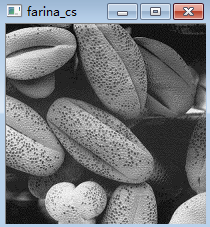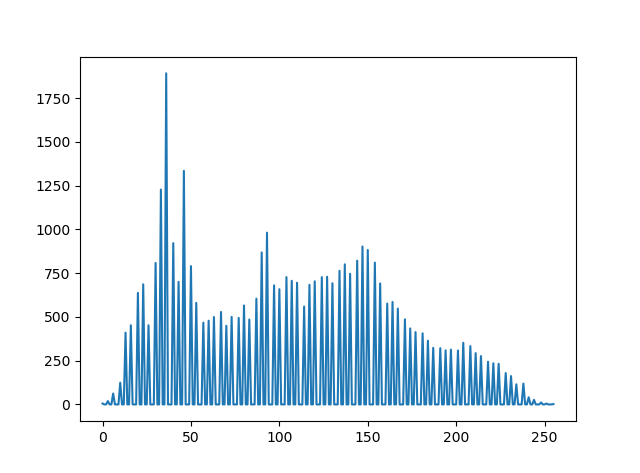$I\left(x,y\right)=I\left(x,y\right)+offset$

farina_cs = farina + 100
cv2.imshow("farina_offset", farina_cs.astype("uint8"))
cv2.waitKey()
hist_full = cv2.calcHist([farina_cs.astype("uint8")], , None, , [0, 256])
plt.plot(hist_full)
plt.show()